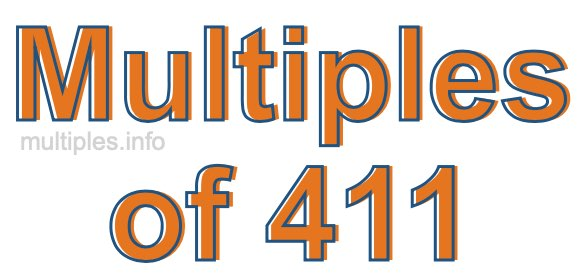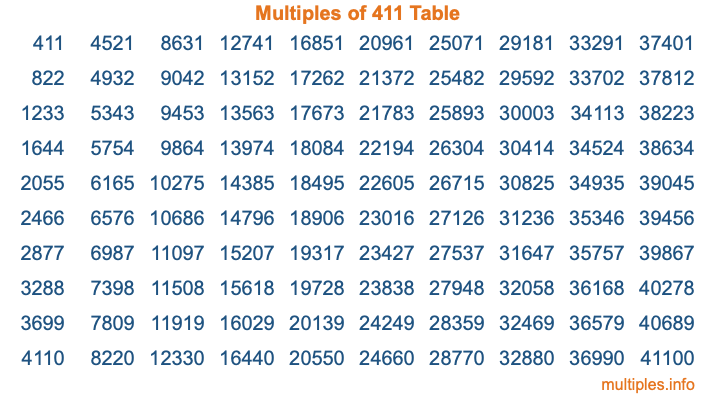Multiples of 411Welcome to the Multiples of 411 page. Here we will first teach you everything you will ever need to know about the multiples of 411, and then give you a study guide summary of everything we taught you to make sure you remember it all. Use this page to look up facts and learn information about the multiples of 411. This page will make you a multiples of four hundred eleven expert!

Definition of Multiples of 411
Multiples of 411 are all the numbers that when divided by 411 equal an integer. Each of the multiples of 411 are called a multiple. A multiple of 411 is created by multiplying 411 by an integer.

Therefore, to create a list of multiples of 411, you start with 1 multiplied by 411, then 2 multiplied by 411, then 3 multiplied by 411, and so on for as long as you want. Thus, the list of the first five multiples of 411 is 411, 822, 1233, 1644, and 2055. To see a larger list of multiples of 411, see the printable image of Multiples of 411 further down on this page. We also have a category where you can choose any nth multiple of 411.

Multiples of 411 Checker
The Multiples of 411 Checker below checks to see if any number of your choice is a multiple of 411. In other words, it checks to see if there is any number (integer) that when multiplied by 411 will equal your number. To do that, we divide your number by 411. If the the quotient is an integer, then your number is a multiple of 411.

Is  a multiple of 411?

Least Common Multiple of 411 and ...
A Least Common Multiple (LCM) is the lowest multiple that two or more numbers have in common. This is also called the smallest common multiple or lowest common multiple and is useful to know when you are adding our subtracting fractions. Enter one or more numbers below (411 is already entered) to find the LCM.

Check out our LCM Calculator if you need more details about the Least Common Multiple or if you need the LCM for different numbers for adding and subtraction fractions.

nth Multiple of 411
As we stated above, 411 is the first multiple of 411, 822 is the second multiple of 411, 1233 is the third multiple of 411, and so on. Enter a number below to find the nth multiple of 411.

th multiple of 411

Multiples of 411 vs Factors of 411
411 is a multiple of 411 and a factor of 411, but that is where the similarities end. All postive multiples of 411 are 411 or greater than 411. All positive factors of 411 are 411 or less than 411.

Below is the beginning list of multiples of 411 and the factors of 411 so you can compare:

Multiples of 411: 411, 822, 1233, 1644, 2055, etc.

Factors of 411: 1, 3, 137, 411

As you can see, the multiples of 411 are all the numbers that you can divide by 411 to get a whole number. The factors of 411, on the other hand, are all the whole numbers that you can multiply by another whole number to get 411.

It's also interesting to note that if a number (x) is a factor of 411, then 411 will also be a multiple of that number (x).

Multiples of 411 vs Divisors of 411
The divisors of 411 are all the integers that 411 can be divided by evenly. Below is a list of the divisors of 411.

Divisors of 411: 1, 3, 137, 411

The interesting thing to note here is that if you take any multiple of 411 and divide it by a divisor of 411, you will see that the quotient is an integer.

Multiples of 411 Table
Below is an image of the first 100 multiples of 411 in a table. The table is in chronological order, column by column. The first column has the first ten multiples of 411, the second column has the next ten multiples of 411, and so on.The Multiples of 411 Table is also referred to as the 411 Times Table or Times Table of 411. You are welcome to print out our table for your studies.

Negative Multiples of 411
Although not often discussed or needed in math, it is worth mentioning that you can make a list of negative multiples of 411 by multiplying 411 by -1, then by -2, then by -3, and so on, to get the following list of negative multiples of 411:

-411, -822, -1233, -1644, -2055, etc.

Multiples of 411 Summary
Below is a summary of important Multiples of 411 facts that we have discussed on this page. To retain the knowledge on this page, we recommend that you read through the summary and explain to yourself or a study partner why they hold true.

There are an infinite number of multiples of 411.

A multiple of 411 divided by 411 will equal a whole number.

411 divided by a factor of 411 equals a divisor of 411.

The nth multiple of 411 is n times 411.

The largest factor of 411 is equal to the first positive multiple of 411.

411 is a multiple of every factor of 411.

411 is a multiple of 411.

A multiple of 411 divided by a divisor of 411 equals an integer.

411 divided by a divisor of 411 equals a factor of 411.

Any integer times 411 will equal a multiple of 411.

Multiples of a Number
Here you can get the multiples of another number, all with the same attention to detail as we did for multiples of 411 on this page.

Multiples of
Multiples of 412
Did you find our page about multiples of four hundred eleven educational? Do you want more knowledge? Check out the multiples of the next number on our list!